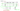## Normal Equation for optimization

Like gradient descent, "Normal Equation" method is another way of minimizing $J$. In the "Normal Equation" method, we will minimize $J$ by explicitly taking its derivatives with respect to the $θ_j$’s, and setting them to zero. This allows us to find the optimum theta without iteration.

The cost function is:

$J(\theta) = \dfrac {1}{2m} \displaystyle \sum _{i=1}^m \left (h_\theta (x^{(i)}) - y^{(i)} \right)^2$

Find $\theta \in \mathbb{R}^{n+1}$, which minimizes cost function $J$: $\frac{\partial}{\partial \theta_j} J(\theta) = 0$ (for every $j \in 0,1,...,n$)

Solve for $\theta_0, \theta_1, ..., \theta_n$$n$ features;
$m$ training examples: $(x^{(1)},y^{(1)}), ..., (x^{(m)},y^{(m)})$

$\space x^{(i)} = \begin{bmatrix} x^{(i)}_0 \\ x^{(i)}_1 \\ x^{(i)}_2 \\ \vdots \\ x^{(i)}_n \end{bmatrix} \in \mathbb{R}^{n+1} \space; \space X = \begin{bmatrix} ... \space (x^{(1)})^T \space ... \\ ... \space (x^{(2)})^T \space ... \\ \vdots \\ ... \space (x^{(m)})^T \space ... \end{bmatrix}$ $($m x (n+1) matrix$)$

$y = \begin{bmatrix} y^{(1)} \\ y^{(2)} \\ \vdots \\ y^{(m)} \end{bmatrix} \in \mathbb{R}^{m}$

The normal equation formula is given below:

$\theta = (X^T X)^{-1}X^T y \space \space \in \mathbb{R}^{n+1}$

Octave/MATLAB Implementation:

pinv(X' * X) * X' * y

NOTE:
There is no need to do feature scaling with the normal equation.

The following is a comparison of gradient descent and the normal equation:

Need to choose learning rate $\alpha$No need to choose $\alpha$
$O(kn^2)$$O(n^3)$, need to calculate inverse of $X^TX$
Works well even if $n$ is largeSlow if $n$ is very large
In practice, when $n$ exceeds 10,000 it might be a good time to go from a normal equation solution to an iterative process.
When implementing the normal equation in Octave/MATLAB, we want to use the pinv function rather than inv. The pinv function will give you a value of $\theta$ even if $X^TX$ is not invertible.
If $X^TX$ is non-invertible, the common causes might be having: